﻿ Sarma | Polygonal Slip Surface | GEO5 | Online nápověda

Odkaz jsme Vám odeslali na email.

Nepodařilo se nám odeslat odkaz na váš email. Zkontrolujte, prosím, Váš email.

# Online nápověda

Stromeček
Nastavení
Produkt:
Program:
Jazyk:

## Sarma

The Sarma method falls within a category of general sliced methods of limit states. It is based on fulfilling the force and moment equilibrium conditions on individual blocks. The blocks are created by dividing the soil region above the potential slip surface by planes, which may have in general experience a different inclination. Forces acting on individual blocks are displayed in the following figure.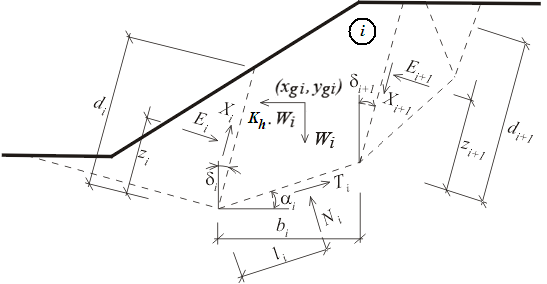Static scheme - Sarma method

Here, Ei ,Xi represent the normal and shear forces between the blocks. Ni ,Ti  are normal and shear forces on segments of a slip surface. Wi is the block weight and Kh*Wi  is the horizontal force that is used to achieve in the Sarma method the limit state. Generally, inclined surcharge can be introduced in each block. This surcharge is included in the analysis together with the surcharge due to water having the free water table above the terrain, and with forces in anchors. All these forces are projected along the horizontal and vertical directions, which are then summed up into components Fxi and Fyi.

Kh is a constant named the factor of horizontal acceleration and it is introduced into the analysis in order to satisfy the equilibrium on individual blocks. There is a relationship between Kh and the factor of slope stability SF allowing for the safety factor computation. In ordinary cases, the analysis proceeds with the value of Kh equal to zero. A non-zero value of Kh is used to simulate the horizontal surcharge, e.g. due to earthquake (see below).

## Analysis process

Computation of limit equilibrium

The computation of limit equilibrium requires the solution of 6n - 1 unknowns, where n stands for the number of blocks dividing the soil region above the potential slip surface. These are:

 Ei - forces developed between blocks Ni - normal forces acting on the slip surface Ti - shear forces acting on the slip surface Xi - shear forces developed between blocks zi - locations of points of applications of forces li - locations of points of applications of forces Kh - factor of horizontal acceleration

5n - 1 equations are available for the required unknowns. In particular, we have:

a)horizontal force equations of equilibrium on blocks: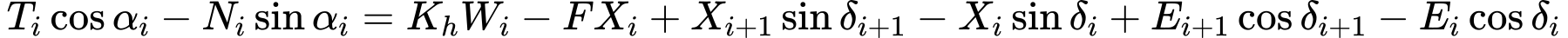b)vertical force equations of equilibrium on blocks: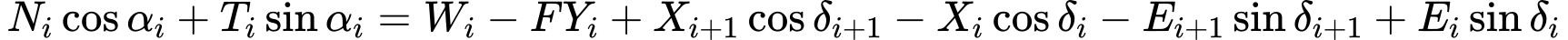c) moment equations of equilibrium on blocks:where rxi and ryi are arms of forces Fxi and Fyi

d) relationship between the normal and shear forces according to the Mohr-Coulomb theory: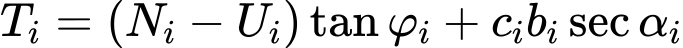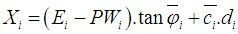where: P*Wi - resultant force of pore pressure on dividing planes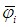- average value of internal friction angle on a dividing plane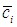- average value of cohesion on a dividing plane

It is evident that n - 1 must be selected (estimated) a priori. A relatively small error is received when estimating the points of application of forces Ei. The problem then becomes statically determined. Solving the resulting system of equations finally provides the values of all remaining unknowns. The principal result of this analysis is the determination of the factor of horizontal acceleration Kh.

Computation of factor of slope stability SF

The factor of slope stability SF is introduced in the analysis such as to reduce the soil strength parameters c and tgφ. Equilibrium analysis is then performed for the reduced parameters to arrive at the factor of horizontal acceleration Kh pertinent to a given factor of slope stability SF. This iteration is repeated until the factor Kh reaches either zero or a specified value.